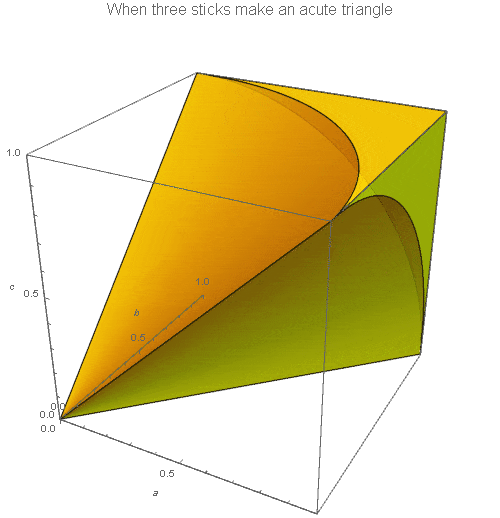# Sticks in the woodsThis Riddler puzzle is about making triangles out of sticks! Here is the problem:

Here are four questions about finding sticks in the woods, breaking them, and making shapes:

1. If you break a stick in two places at random, forming three pieces, what is the probability of being able to form a triangle with the pieces?
2. If you select three sticks, each of random length (between 0 and 1), what is the probability of being able to form a triangle with them?
3. If you break a stick in two places at random, what is the probability of being able to form an acute triangle — where each angle is less than 90 degrees — with the pieces?
4. If you select three sticks, each of random length (between 0 and 1), what is the probability of being able to form an acute triangle with the sticks?

For the tl;dr, here are the answers:
[Show Solution]

Here are detailed solutions to all four problems (with cool visuals!):
[Show Solution]

## 2 thoughts on “Sticks in the woods”

1.Hector Pefo says:

Let there be $n$ breaks.

Lay the pieces down in original order, left to right. Now consider one of the breaks, $B$. $B$ is the break to the right of a piece of length greater than or equal to $1/2$ just in case $B$ is greater than $1/2$ (probability $1/2$) and all of the other $n-1$ breaks are outside of the region between $B$ and the point $1/2$ to its left (probability $1/2^{n-1}$). So $B$’s probability of being such a break is $1/2^n$. Since each of the $n$ breaks has that chance of being to the right of a longer-than-$1/2$ piece, and so does the very right end of the stick, and no more than one of these can be, in all the chance that one of them does so is $(n+1)/2^n$. Therefore the chance that no break is to the right of such a piece, and so we can form a $(n+1)$-gon, is $1-(n+1)/2^n$.

2.Spreck Rosekrans says:

Laurent:

I’m the one who sent these questions to the Riddler. The first one was posed when I was in college, and then I thought up the other questions as well as the one Hector mentioned above (though it my answer was far longer) – sometime in the late 70’s or early 80’s.

The best part about the answers for the acute triangles in my view is that they both depend on conic sections – one with pi and one with e.

I really enjoyed the nice graphics. I have imagined those shapes, especially the last one, for some time. I don’t know how to do this stuff.

Best,

Spreck